8.2. Calculation of the Spectrum of the Hydrogen Atom

Let us insert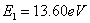and n=2, 3, 4… into the formulas (206) and (207). As a result, we’ll have the theoretical values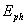(theor.) of energies of the photons being absorbed or emitted by the electron during its energy transitions in the hydrogen atom, which practically completely coincide with the experimental values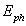(exper.) of these energies and binding energy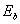of this electron with the atomic nucleus (Table 5).

Table 5.  Spectrum of the hydrogen atom

 Volumes n 2 3 4 5 6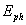(exper) eV 10.2 12.09 12.75 13.05 13.22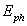(theor) eV 10.198 12.087 12.748 13.054 13.22(theor.) eV 3.4 1.51 0.85 0.54 0.38

It appears from the law of spectroscopy (207) that energies of the photons being absorbed and emitted during the transition of the electron between the energy levels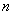and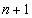are calculated according to the formula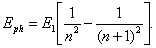(216)

Let us give the results of the calculation (Table 6) according to this formula of the energies of the photons(theor.) being emitted or absorbed by the electron of the hydrogen atom  at the interlevel transitions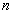andas compared with the experimental(exper.) data.

Table 6. Energies of interlevel transitions of the electron of the hydrogen atom

 Levels n, n+1 2...3 3...4 4...5 5...6 6...7 7...8(exper) eV 1.89 0.66 0.30 0.17 0.10 0.07(theor) eV 1.888 0.661 0.306 0.166 0.100 0.065

Let us note that binding energiesof the electron with the atomic nucleus are given in Table 5. The rest energies of the photons being emitted or absorbed during the energy transitions of the electron are given in Tables 5 and 6.

The formula (216) gives the possibility to calculate the energies of the photons being absorbed and emitted during any energy transition of the electron. For example, when the electron goes from the third to the tenth energy level, it absorbs the photon with the energy, which is calculated according to the formula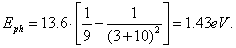(217)

If the electron goes from the fifteenth to the fifth energy level, it emits the photon with the energy of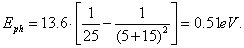(218)

Thus, these formulas give the possibility to calculate energy of the absorbed and emitted photon by the electron during its transition between any energy levels.

8.3. Calculation of the Spectra of the Hydrogen-like Atoms

The atoms, which have one electron, are called the hydrogen-like atoms. We have shown that binding energy of the hydrogen atom at the time of its stay on the first energy level is equal to ionization energy of this atom. The same regularity is observed with all hydrogen-like atoms, i.e. the atoms, which have one electron , , .

As in the atoms, which have many electrons,  the electrons having the largest ionization energy are the nearest electrons to the atomic nucleus, we have called them the first electrons in our previous publications. Our further investigations have shown that this numeration makes the further mathematical models for the calculation of the spectra of the atoms with many electrons more complicated, we return to the numeration of the electrons, which originates from the numeration of the potentials of ionization of the atoms and ions , , , , , , , , , , , .

For example, the helium atom has two electrons. One has ionization energy 54.416 eV and another 24.587 eV. Taking into consideration the above-mentioned facts we’ll consider the electron with less ionization energy of 24.587 eV to be the first electron of the helium atom and the electron with larger energy of 54.416 eV to be the second one. It means that lithium, the next element, will have the electron with ionization energy of 5.392 eV to be the first one, the electron with ionization energy of 75.638 eV to be the second one and the electron of 122.451 eV to be the third one. We’ll accept similar numeration of the electrons for other chemical elements.

It is known that the number of chemical element Z in Mendeleyev’s law corresponds to the number of the protons in the atomic nucleus, and binding energy of the electron of the hydrogen-like atom at the time of its stay on the first energy level is proportional to square of the number of the protons in the nucleus.

Binding energy of the electron with the proton (nucleus) of the hydrogen atom, which corresponds to the first energy level, is equal to 13.598 eV. It means that binding energy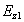of the electron of the hydrogen-like atom of any other element corresponding to the first energy level, will be equal to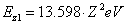(219)

The theoretical and experimental values of binding energies of the electrons of the hydrogen-like atoms, which correspond to their first energy levels, are given for some chemical elements below (Table 7).

Table 7. Theoretical and experimental values of binding energies of the electrons of the hydrogen-like atoms, which correspond to their first energy levels

 Chemical elements Number of element Z Binding energies, eV experiment theory H 1 13.598 - He 2 54.416 54.392 Li 3 122.451 122.382 Be 4 217.713 217.568 B 5 340.217 339.950 C 6 489.981 489.528 N 7 667.029 666.302 O 8 - 870.272

At present, it is considered that with the increase of the number of the protons in the nucleus the number of the neutrons is increased as well as the mass of the nucleus. It causes the increase of gravitational forces influencing the electron. As a result, it is supposed that the gravitational forces begin to influence the processes of absorption and emission of the photons, but the relations (219) does not take it into account. That’s why the divergence between the experimental values of binding energies of the electrons of the hydrogen-type atoms and the results of the theoretical calculations (Table 7). But later on we’ll see that the reason of this divergence is different.

Let us introduce a very important notion. Let us suppose that a stationary energy level of the electron in the atom is such energy level, on which the electron can absorb such photon when its binding energy with the nucleus becomes equal to zero, and it will become free. Then binding energies of the electrons of the hydrogen-like atoms corresponding to stationary energy levels will be calculated according to the formula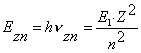.                                                       (220)

Symbol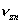denotes the own frequency of the photon emitted by the electron when it moves from the stationary energy level n to free state.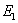=13.598 eV is ionization energy of hydrogen atom. The results of the calculation according to the formula (220) are given in Table 8.

Table 8. Theoretical and experimental values of binding energies of the electrons with nuclei of the hydrogen-like atoms corresponding to the stationary energy levels

 Z Ele-ment Determination method Energies of stationary levels, eV n=1 n=2 n=3 n=4 1 H Exper. Theor. 13.598 - 3.398 3.399 1.508 1.511 0.848 0.849 2 He Exper. Theor. 54.416 - 13.606 13.604 6.046 6.046 3.396 3.401 3 Li Exper. Theor. 122.451 - 30.611 30.613 13.601 13.607 7.651 7.653 4 Be Exper. Theor. 217.713 - 54.423 54.428 24.183 24.190 13.613 13.607 5 B Exper. Theor. 340.217 - 85.047 85.054 37.797 37.801 21.257 21.263 6 C Exper. Theor. 489.981 - 122.461 122.495 54.431 54.446 30.611 30.624 7 N Exper. Theor. 667.029 - 166.689 166.757 74.089 74.114 41.669 41.689

We’ll not calculate the spectra of the electrons of the hydrogen-like atoms of all  chemical elements. Let us give only the method of the calculation for some of them. For example, let us calculate the spectrum of the electron of the hydrogen-like atom of lithium (the third one) corresponding to its second stationary energy level. The third electron of this atom has the largest ionization energy. It is equal to binding energy of this electron with the atomic nucleuscorresponding to the first energy level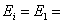122.451 eV. If we insert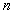=2, 3, 4, ……. in the formulas (206) and (207), we’ll find (Table 9).

Table 9. Spectrum of the third electron of the lithium atom and its binding energieswith the atomic nucleus on the stationary energy levels

 Values n 2 3 4 5 6(exper.) eV 91.84 108.84 114.8 117.55 119.05(theor.) eV 91.84 108.85 114.8 117.55 119.05(theor.) eV 30.61 13.6 7.65 4.8 3.4

The relation (207) was called by us a law of formation of the spectra of the atoms and the ions, because before the determination of this law the spectra of hydrogen-like atoms were calculated with the help of Balmer-Rydberg’s equation or Schroedinger’s equation, and in order to calculate the spectra of all further electrons, the approximate numerical methods were used . The equation (207) gives the possibility to calculate the spectra of all electrons, but under certain conditions. Let us consider these conditions in detail taking the calculation of the spectrum of the first electron of helium atom as an example.

8.4. Calculation of the Spectrum of the Helium Atom

The helium atom has two electrons. Ionization energy of the first one is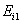=24.587 eV, and ionization energy of the second one is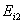=54.416 eV .

The state of the helium atom when both its electrons are on the first energy levels is called the main unexcited one. The second electron remains on the first energy level till the first electron remains in the atom. Once the first electron leaves the atom, the second electron passes to an excited state. Excitation energy is the energy of the absorbed photon. It is equal to the difference between ionization energy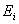of the electron and binding energy of the electron with the atomic nucleus corresponding to the energy level, to what the electron passes after the photon is absorbed. We call such levels the stationary ones.

Taking into consideration the above-mentioned mentioned facts, let us present a row of the stationary energy levels of the second electron of the helium atom and binding energies of the electron with the nucleus corresponding to them and excitation energies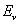(Table 10) , , .

Table 10. Energy indices of stationary energy levels of the second electron of the helium atom

 Number of the level, n Binding energy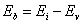, eV Excitation energies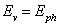, eV 1 54.416 0.00 2 13.60 40.81 3 6.09 48.37 4 3.39 51.02 5 2.17 52.24 6 1.51 52.90 7 1.11 53.31 8 0.85 53.57 9 0.67 53.74 10 0.55 53.87 11 0.45 53.97 12 0.38 54.04 … … … - 0.00 54.416

The helium atom with one electron is in ionized state, that’s why it is called a helium ion. We have already shown that regularity of the change of the energies of the stationary energy levels is the same with all atoms, which consist of a nucleus and one electron. The spectra of such ions are calculated according to the mathematical model (207) of the law of formation of the spectra of the atoms and the ions .

We’d like to draw the attention of the readers to the main fact: the possibilities of Schroedinger’s equation concerning the spectrum calculation are finished with hydrogen-like atoms. Schroedinger’s equation does not give an opportunity to calculate the spectrum of the first electron of helium atom. Let us consider the possibility of our mathematical model (207) of formation of the spectra of the atoms and the ions to calculate the spectrum of the first electron of the helium atom.

For this purpose, let us write from the reference book  the excitation energies of the first electron of the helium atom, which correspond to the stationary energy levels. At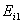=24.587 eV we have (Table 11).

Table 11. Energy indices of stationary energy levels of the first electron of the helium atom

 Number of the level, n Binding energy, eV Excitation energies, eV 1 ? ? 2 3.627 20.96 3 3.367 21.22 4 1.597 23.01 5 1.497 23.09 6 0.847 23.74 7 0.547 24.04 8 0.377 24.21 9 0.277 24.31 10 0.217 24.37 11 0.167 24.42 12 0.137 24.45 13 0.117 24.47 14 0.097 24.49 15 0.077 24.51 16 0.067 24.52 … ? 59.67

We’d like to remind that binding energiesof the first electron with the atomic nucleus are determined as the difference between the ionization energy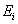=24.587 eV and the excitation energies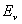being equal to the energies of the absorbed photons or emitted photons(Table 11).

The last line in Table 8 looks abnormal. Why the maximal excitation energy of 59.67 eV is larger than ionization energy not only of the first electron (=24.587 eV), but of the second one (=54.416 eV)? It is not simple to get the answer to this question, but we’ll try to formulate the first hypothetical explanation of the noted anomaly later on.

The accuracy of the experimental data of spectroscopy plays a decisive role in our search, because we’ll form further notions concerning a picture of the interaction of the first electron with the nucleus of the helium atom on their basis.

We’d like to note that in the reference books  and  the experimental values of ionization energies are determined with the accuracy up to the third character, and the excitation energies of the stationary energy levels are determined with the accuracy up to the second character. The writers of the reference books note that the value of the second character of excitation energies differs with various authors by ±0.02 eV and sometimes even more.

Consequently, in order to determine an empirical law, which forms a series of excitation energies of the stationary energy levels of the first electron of the helium atom, it is necessary to round the results of the calculations of binding energies to the second character after point.

It is not all that should be taken into consideration when analyzing the experimental data. Let us pay attention to the following sentences in the reference book : “Together with the experimentally measured wavelengths, there are such lines in the tables being suggested, which wavelengths are calculated according to the energy levels taking into consideration the selection regulations. These are either the fine structure lines, which are not yet discovered, or weak, rough measured spectral lines. Validity of such calculation makes no doubts, because energy levels are established according to the safely measured lines with the implementation of the secondary standards”.

It is difficult to agree with such method of the experimenters. Let us consider excitation energy of 23.01 eV (Table 11), which corresponds to the fourth stationary energy level. It is not available in the reference book ; in the reference book  it is given without a line brightness designation, i.e. as a very weak or unobserved. What should we do in this case? It is wise not to take it into consideration for the time being during the search of regularity of formation of excitation energies, which correspond to the stationary energy levels. Excitation energy being equal to 20.96 eV is in the same position. That’s why let us exclude it from our consideration.

At once, let us pay attention to the fact how they have got the empirical law for the calculation of binding energies of the first electron of helium atom given in Table 12,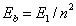coinciding completely with the law (206), which forms binding energies of the electrons of hydrogen-like atoms. For this purpose, energy of 3.37 eV corresponding to excitation energy of 21.22 eV in Table 11 was taken and multiplied by 4. The number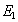=13.468 eV being obtained was the binding energy corresponding to the first energy level of the first electron of the helium atom. Of course, it is  fictitious energy, but a variety of energies formed in this case (Table 12, the last column) coincides completely with a variety of the corresponding experimental values confirming competence of exclusion of excitation energies of 20.96 eV and 23.01 eV from this variety. The result being obtained shows that binding energy of the fist electron of helium atom corresponding to the first energy level of=13.468 eV is not equal to ionization energy of this electron=24.587 eV.

Table 12. Binding energiesof the first electron of the helium atom with its nucleus being determined experimentally and theoretically

 Energy level number, n Excitation energy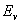eV Energy of binding,  eV experiment theory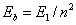1 24.586 ? 13.47 2 21.22 3.37 3.37 3 23.09 1.50 1.50 4 23.74 0.85 0.85 5 24.04 0.55 0.55 6 24.21 0.38 0.38 7 24.31 0.28 0.28 8 24.37 0.22 0.22 9 24.42 0.17 0.17 10 24.45 0.14 0.14 11 24.47 0.10 0.10 12 24.49 0.09 0.09 13 24.51 0.08 0.08 14 24.52 0.07 0.07

The results of this Table demand a return to the experiment connected with the determination of the spectrum of the first electron of helium atom in order to determine availability of lack of experimental lines, which correspond to energies 20.96 eV and 23.01 eV.

A question arises: why do the values of ionization energyand binding energywith the nucleus corresponding to the first energy level of the second electron of the helium atom coincide (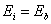=54.416 eV), and the values of the first electron fail to coincide (=24.587 eV and=13.468 eV)? We have failed to find the reply to this fundamental question, and we address it to the future investigators.

An interesting and chargeable moment comes. If the mathematical model (207) is in fact a law of formation of the spectra of the atoms and the ions, we should get the experimental values of excitation energies corresponding to the stationary energy levels with its help. If we insert=24.587 and=13.468 into the formulas (206) (207), we’ll get (Table 13).

Table 13. Spectrum of the first electron of the helium atom

 Valumes n 2 3 4 5 6(exper) eV 21.22 23.09 23.74 24.04 24.21(theor.) eV 21.22 23.09 23.74 24.05 24.21(theor.) eV 3.37 1.5 0.84 0.54 0.37

Then we’ll get the spectra of other atoms with many electrons using the determination method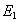of binding of the electron with the nucleus corresponding to the fist energy level worked out with the implementation of the analysis of the spectrum of the first electron of the helium atom.

The Foundations of Physchemistry of Microworld

Ó2003 Kanarev Ph. M.

Internet Version - http://book.physchemistry.innoplaza.net

<< Back to Physchemistry Book Index